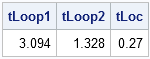The SAS/IML language is a vector language, so statements that operate on a few long vectors run much faster than equivalent statements that involve many scalar quantities.

For example, in a previous post, I asserted that the LOC function is much faster than writing a loop, for finding observations that satisfy some criteria. It is easy to use the TIME function in Base SAS software to time how long (in seconds) a SAS/IML computation takes.

Suppose you have one million observations and five variables in a matrix:

```proc iml; x = j(1e6, 5); call randgen(x, "Normal"); /** each var is random normal **/```

Suppose you want to find all the observations which have positive values for all variables. There are several ways to do this, and some are more efficient than others.

One approach, which is not efficient, is to loop over the one million observations and test each of the five variables. The following loop performs five million scalar comparisons:

```/** element-by-element comparison: VERY SLOW! **/ free ndx; t0 = time(); /** starting time **/ do i = 1 to nrow(x); if (x[i,1] > 0 & x[i,2] > 0 & x[i,3] > 0 & x[i,4] > 0 & x[i,5] > 0) then ndx = ndx || i; /** resize the result: SLOW **/ end; tLoop1 = time() - t0; /** elapsed time **/```

Alternatively, you could loop over the one million observations and test whether the ith row is a positive vector. This technique requires one million comparisons; each comparison involves a (short) vector.

```/** row-by-row comparison **/ free ndx; t0 = time(); do i = 1 to nrow(x); if all(x[i,] > 0) then ndx = ndx || i; /** resize the result: SLOW **/ end; tLoop2 = time() - t0; /** elapsed time **/```

Tip: The performance of both of the previous loops can be improved by allocating the ndx vector outside of the loop instead of using the horizontal concatenation operator (||) to create the vector one element at a time.

A faster way to test these data is to use the LOC function to extract the locations in which each column is positive. This technique requires five comparisons, although each comparison involves a very long vector.

```/** column comparisons **/ t0 = time(); ndx = loc(x[,1] > 0 & x[,2] > 0 & x[,3] > 0 & x[,4] > 0 & x[,5] > 0); tLoc = time() - t0;/** elapsed time **/   print tLoop1 tLoop2 tLoc;```Clearly, the first technique is the big loser. The second technique is 2.3 times faster than the first. The LOC technique is almost 16 times faster than the first technique. However, the second technique does not require that you know how many columns are in the data matrix, whereas the third technique does. (Although this deficiency can be rectified.) And, as I mentioned, you can speed up the first two computations by preallocating the ndx vector instead of using horizontal concatenation within the loop.

Can you do better? Given a data matrix (of any dimension), can you write an efficient module that finds the rows for which all elements are positive? Test the module on matrices of different shapes, such as 1e6 x 5, 5e5 x 10, and 2.5e5 x 20.

By the way, "LOC" rhymes with "joke," which leads to the following limerick:

There once was a bloke from Bear Oak
Who looped when he ought to have LOC-ed.
His terrible gaffe
It was an impractical joke.

ShareDistinguished Researcher in Computational Statistics

Rick Wicklin, PhD, is a distinguished researcher in computational statistics at SAS and is a principal developer of SAS/IML software. His areas of expertise include computational statistics, simulation, statistical graphics, and modern methods in statistical data analysis. Rick is author of the books Statistical Programming with SAS/IML Software and Simulating Data with SAS.

1.Dale McLerran on

Indeed, it is possible to achieve performance which improves significantly on the column comparisons. We can find the location of all positive minimum values returned by a subscript reduction operator. So, we can use

/** location of row min GT 0 **/
t0 = time();
ndx = loc(x[ ,>< ] >0);
tloc2 = time() - t0;

For matrices of the various dimensions suggested, the method which I suggest is three to four times faster than the column comparisons process (with time specified by tloc).

2.Hi Rick,

In line with using subscript reduction operators, I would like to dodge looping over the following scenario:

I have a longitudinal dataset (hypothetical) as below with 4 variables
(id, gender, time and y);

id gender time y
1 0 1 0.2
1 0 2 1.4
1 0 3 0.9
2 1 1 2.3
2 1 2 7.8
2 1 3 0.1
2 1 4 1.7;

I would like to EFFICIENTLY (because the datasets can get very large)
create pairwise combinations, in SAS/IML or using a datastep, within
id for the variables gender and y (number of variables can vary) so
that I have a final dataset with variables id, pair (which is the
combination based on the variable time. E.g., id=1 appears 3 times,
then the possible pair combinations (s,t) are 1,2; 1,3; and 2,3),
gender_s, gender_t, y_s and y_t. The final dataset would look as
follows:

id pair gender_s gender_t y_s y_t;
1 (1,2) 0 0 0.2 1.4
1 (1,3) 0 0 0.2 0.9
1 (2,3) 0 0 1.4 0.9
2 (1,2) 1 1 2.3 7.8
2 (1,3) 1 1 2.3 0.1
2 (1,4) 1 1 2.3 1.7
2 (2,3) 1 1 7.8 0.1
2 (2,4) 1 1 7.8 1.7
2 (3,4) 1 1 0.1 1.7

Kindly advise on how best (in terms of efficiency) I can program this?